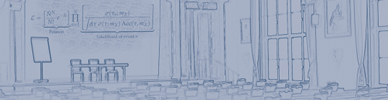#hadron2011

Jun 13 – 17, 2011
Künstlerhaus
Europe/Berlin timezone
Proceedings are now available online at <a href="http://www.slac.stanford.edu/econf/C110613/">eConf</a>

## A new interpretation for the $D^*_{s2}(2573)$ and the prediction of novel exotic charmed mesons

Jun 13, 2011, 5:10 PM
20m
Millerzimmer (Künstlerhaus)

### Speaker

Mrs Raquel Molina (IFIC)

### Description

We study the vector - vector interaction within the hidden gauge formalism in a coupled channel unitary approach. In the sector $C=1,S=1,J=2$ we get a pole in the T-matrix around $2572$ MeV that we identify with the $D^*_{s2}(2573)$, coupling strongly to the $D^*K^*$($D^*_s\phi$($\omega$)) channels. In addition we obtain resonances in other exotic sectors which have not been studied before such as $C=1,S=-1$, $C=2,S=0$ and $C=2,S=1$. This 'flavor-exotic' states are interpreted as $D^*\bar{K^*}$, $D^*D^*$ and $D^*_sD^*$ molecular states but have not been observed yet. In total we obtain nine states with different spin, isospin, charm and strangeness of non $C=0,S=0$ and $C=1,S=0$ character, which have been reported before.

### Primary author

Mrs Raquel Molina (IFIC)

### Co-authors

Prof. Eulogio Oset (IFIC) Mrs Tania Branz (Tuebingen University)

### Presentation materials

 Paper Slides